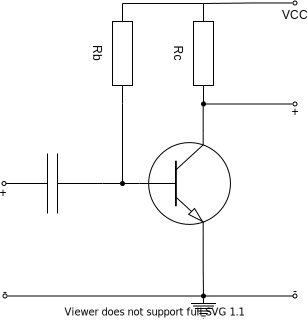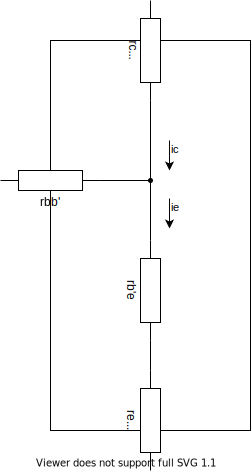# Small-signal Model for BJT

You are at wiki>Analog Electrics>Small-signal Model for BJT

In a Grounded-emitter Amplifier Circuit belowWhen we ignore the existence of AC signal, we have

$\left\{\begin{array}{l}I_{BQ}=\frac{V_{CC}-V_{BEQ}}{R_b}\\I_{CQ}=\beta I_{BQ}\end{array}\right.$

so we can have the static working point Q as

$V_{CEQ}=V_{CC}-I_{CQ}R_c$

based on the output curve, at the working point Q, we can have a small-signal model ONLY FOR AC asIf you find it hard to remember, just remember that base and emitter serves as a resistor and will always be linked with a $r_{be}$, and collecter and emitter serves as a current-controlled source and will be always linked with a power source. As $r_{ce}>>0$ and sometimes even near $\infty$, we can totally ignore it.

Based on the working Q point and the signal source, we can easily have all the other component aside from $r_{be}$, and $r_{be}$ is accually the slope of the output curve($V_{be}-i_{B}$), with what we know about BJTs, we have

$i_C=\beta i_{b}$

so that with KCL we have

$i_e=i_b+i_c=(1+\beta)i_b$

let's take a look at the equivalent resistence in a BJTas the dosage concentration is a lot higher at collecter than emmiter, $r_{b'e}>>r_c$. Also, when we don't take heat loss into account, $r_e$ can be ignored.

As a result, the $r_{be}$ for $i_b$ shold be written as

$r_{be}=r_{bb'}+(1+\beta)r_{b'e}$

we know $r_{bb'}$ for it is a constant for every BJT, so we only need to get $r_{b'e}$, which is the slope for $U_{B'E}-I_e$,As we know what the form of the current on a PN node is, we can have

$I_{e}=I_{ES}(e^{\frac{U_{BE}-I_br_{bb'}}{U_T}}-1)$

based on the fact that $r_{bb'}$ is small compared ro $r_{b'e}$, we can simplify as

$I_{e}=I_{ES}e^{\frac{U_{BE}}{U_T}}$

derivate on both sides, we have

$dI_e=\frac{I_{ES}}{U_T}e^\frac{U_{BE}}{U_T}dU_{BE}$

so that we can have

$r_{b'e}=\frac{dU_{BE}}{dI_e}|_{U_{BEQ}}=\frac{U_T}{I_{EQ}}$

we can have

$r_{be}=r_{bb'}+(1+\beta)\frac{U_T}{I_{EQ}}$

and totally simplify it into a small-signal model.

We can also know the parameters for perfermance, which the input resistence is $R_i=R_b//r_{be}$,the output resistence is $R_o=R_C$, and the amplification is

$A_V=\frac{u_o}{u_i}=\frac{\beta i_BR_C//R_L}{i_Br_{be}}=\frac{\beta R_C//R_L}{r_{be}}$